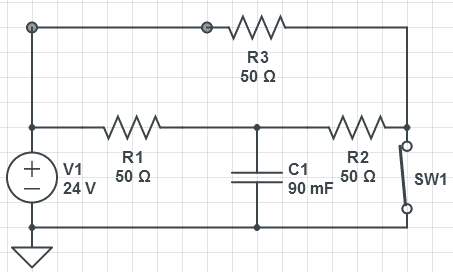# RC Circuits and Time Constants

• Engineering

## Homework Statement

For the circuit below, the switch has been closed for a period of time long enough for steady state conditions to have been reached.a: Determine the time constant before the switch is opened
b: Determine the time constant after the switch is closed
c: Determine the voltage across the capacitor before the switch is opened
d: Determine the voltage across the capacitor after the switch is closed
e: Draw a graph of the voltage vs time, with sufficient time duration that steady state conditions are shown

## Homework Equations

τ=RC
v(t) = V(0)(1-e^(-t/τ)

## The Attempt at a Solution

Here is what I got

a: τ = 33.3Ω * 90mF, τ = 3
note: 33.3Ω is a result of (50*100/150), or (r1 + r2) || r3 as the circuit is flowing through the switch

b: τ = 33.3Ω * 90mF, τ = 3
note: 33.3Ω is a result of (50*100/150), or (r3 + r2) || r1 as the circuit is flowing through the capacitor (until the capacitor becomes an "open circuit")

c: v(t) = ?, not sure what to do here, or how to go about it. I have a formula, but every approach I can think of I either end up with 0, or infinity. Speaking in terms of what happens with the e function, -t/τ. Because when the switch is closed, we can think of t as infinity I think (because the voltage\current relationship will be the same for ever in ideal conditions), so you would get e^ (-infinity/3) --> 0), e^0 = 1, so 24(1-1) = 0.

d: Don't think you even need to mathematically prove that the voltage across the capacitor (as the only "exit" route, as the capacitor charges, current goes to 0 as voltage goes to its limit), so voltage across the open switch circuit would be 24V.

Could use some help here, am I on the right path with my time constants? If not, what am I doing wrong, and what can I do to find c? (probably also what can I do for d, I can't imagine a verbal explanation would satisfy the instructor)

rude man
Homework Helper
Gold Member
a) and b) are the same. So arer c) and d). I wonder if this is a very poor paraphrasing of the given problem ...

gneill
Mentor
a) and b) are the same. So arer c) and d). I wonder if this is a very poor paraphrasing of the given problem ...

Really? I see different equivalent resistances for the two cases.

rude man
Homework Helper
Gold Member
Really? I see different equivalent resistances for the two cases.

"a: Determine the time constant before the switch is opened
b: Determine the time constant after the switch is closed "

"Before the switch is opened" and "after the switch is closed" are not the same thing? Like, in both cases the switch is closed!

Same for c & d.

"a: Determine the time constant before the switch is opened
b: Determine the time constant after the switch is closed "

"Before the switch is opened" and "after the switch is closed" are not the same thing? Like, in both cases the switch is closed!

Same for c & d.

Yeah, I just noticed that myself... Either way, anyone have any advice? Fortunately this is just extra credit for the class, but either way, I would like to be able to figure out what the heck is going on

gneill
Mentor
"a: Determine the time constant before the switch is opened
b: Determine the time constant after the switch is closed "

"Before the switch is opened" and "after the switch is closed" are not the same thing? Like, in both cases the switch is closed!

Same for c & d.

D'Oh! You're right! I parsed right over that and drew rational conclusions rather than right ones. Clearly the devil is in the detailsgneill
Mentor
Yeah, I just noticed that myself... Either way, anyone have any advice? Fortunately this is just extra credit for the class, but either way, I would like to be able to figure out what the heck is going on

Have you covered equivalent (net) resistance? How about Thevenin equivalents? In either position of the switch, what equivalent resistance does the capacitor "see" when the source is suppressed (voltage source replaced by a short circuit)?

rude man
Homework Helper
Gold Member
Yeah, I just noticed that myself... Either way, anyone have any advice? Fortunately this is just extra credit for the class, but either way, I would like to be able to figure out what the heck is going on

Yes, I have some advice. Get the problem defined correctly! :grumpy:

Don't get mad at me! I copied that word for word off my assignment lol!

Have you covered equivalent (net) resistance? How about Thevenin equivalents? In either position of the switch, what equivalent resistance does the capacitor "see" when the source is suppressed (voltage source replaced by a short circuit)?

Yes we have in both.

rude man
Homework Helper
Gold Member
Don't get mad at me! I copied that word for word off my assignment lol!

So then you need to straighten out your instructor or ...